# Algebra 1 : Graphing

## Example Questions

← Previous 1 3 4 5

### Example Question #1 : Functions And Lines

The points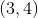and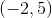are plotted on a quadrant. Which graph depicts the correct points?

Explanation:

A quadrant is set up in such a way that the positive numbers are to the right and top, while the negative numbers are to the left and bottom. The x-value (the first number in the ordered pair) is the distance left or right from the center. The y-value (the second number in the ordered pair) is the distance above or below the axis.will beunits to the right, andunits up.will beunits to the left, andunits up.

### Example Question #2 : Functions And Lines

Which of the followiing quadrants would contain the point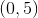?

None of the other answers is correct.

None of the other answers is correct.

Explanation:has 0 as its-cordinate and is therefore on the-axis, which is not part of any of the four quadrants.

### Example Question #3 : Functions And Lines

Which of the following points would be in Quadrant III on the coordinate plane?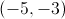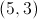None of the points listed would be in Quadrant III.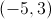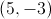Explanation:

Quadrant III comprises the points whose- and- coordinates are both negative. This makes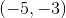the correct choice.

### Example Question #4 : Functions And Lines

Which of the following quadrants would contain the point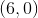?

None of the other answers is correct.

None of the other answers is correct.

Explanation:has 0 as its-coordinate and is therefore on the-axis, which is not part of any of the four quadrants.

### Example Question #1 : Graphing

Which of the following ordered pairs is in the third quadrant?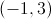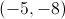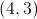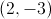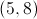Explanation:

The third quadrant is the lower left quadrant, where both theandvalues are negative.  The point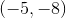is the only point that is in this quadrant.

### Example Question #1 : Functions And Lines

Which of the following points is in Quadrant IV on the coordinate plane?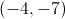Two of these points are in Quadrant IV.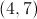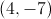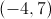Explanation:

Quadrant IV consists of the points with positive-coordinates and negative-coordinates. Therefore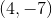is the correct choice.

### Example Question #1 : How To Graph An Ordered Pair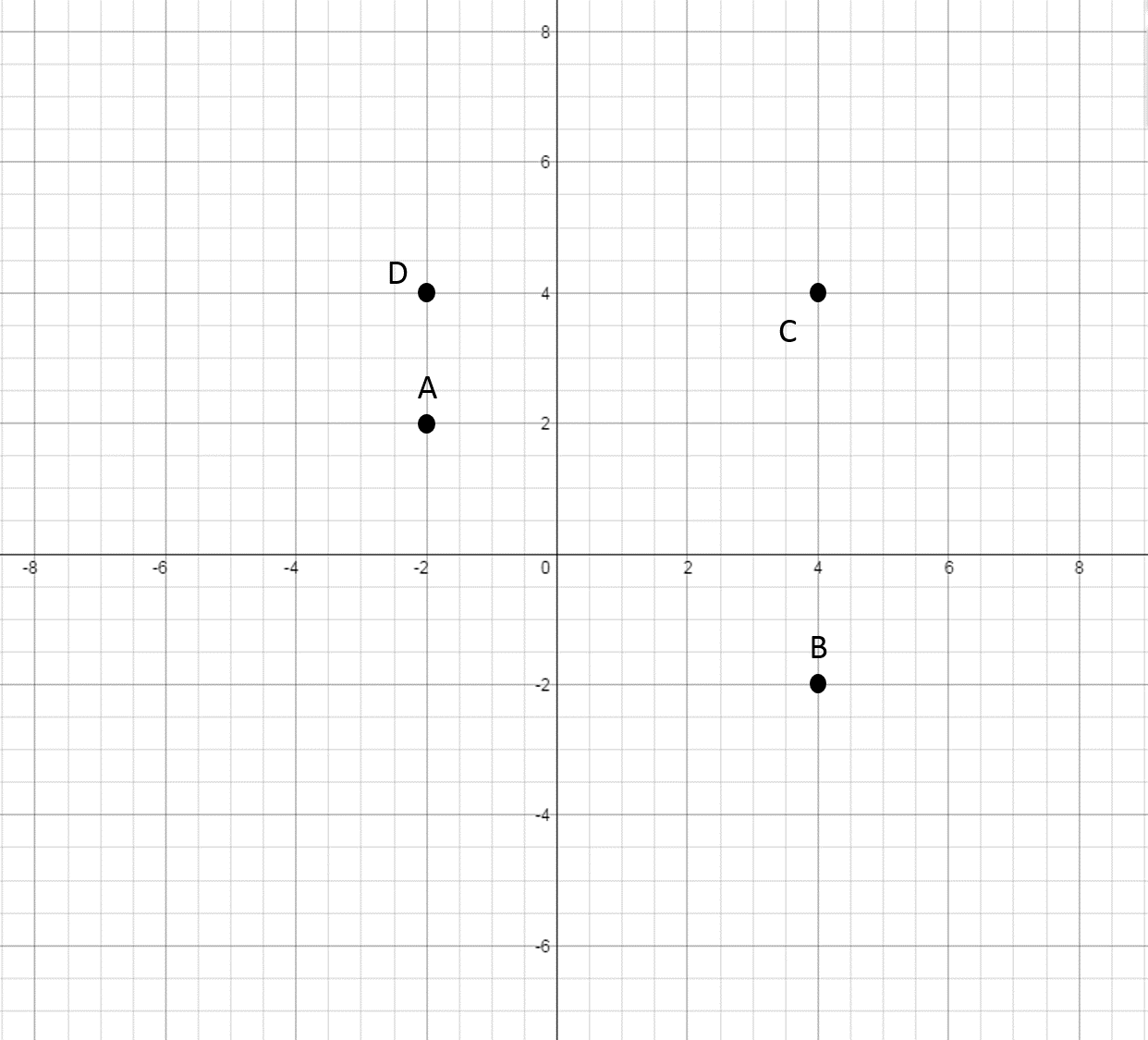Find the point that corresponds to the following ordered pair: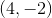Explanation:

In order to get to the point, start at the origin and move rightunits and downunits.

This is point.

### Example Question #1 : How To Graph An Ordered Pair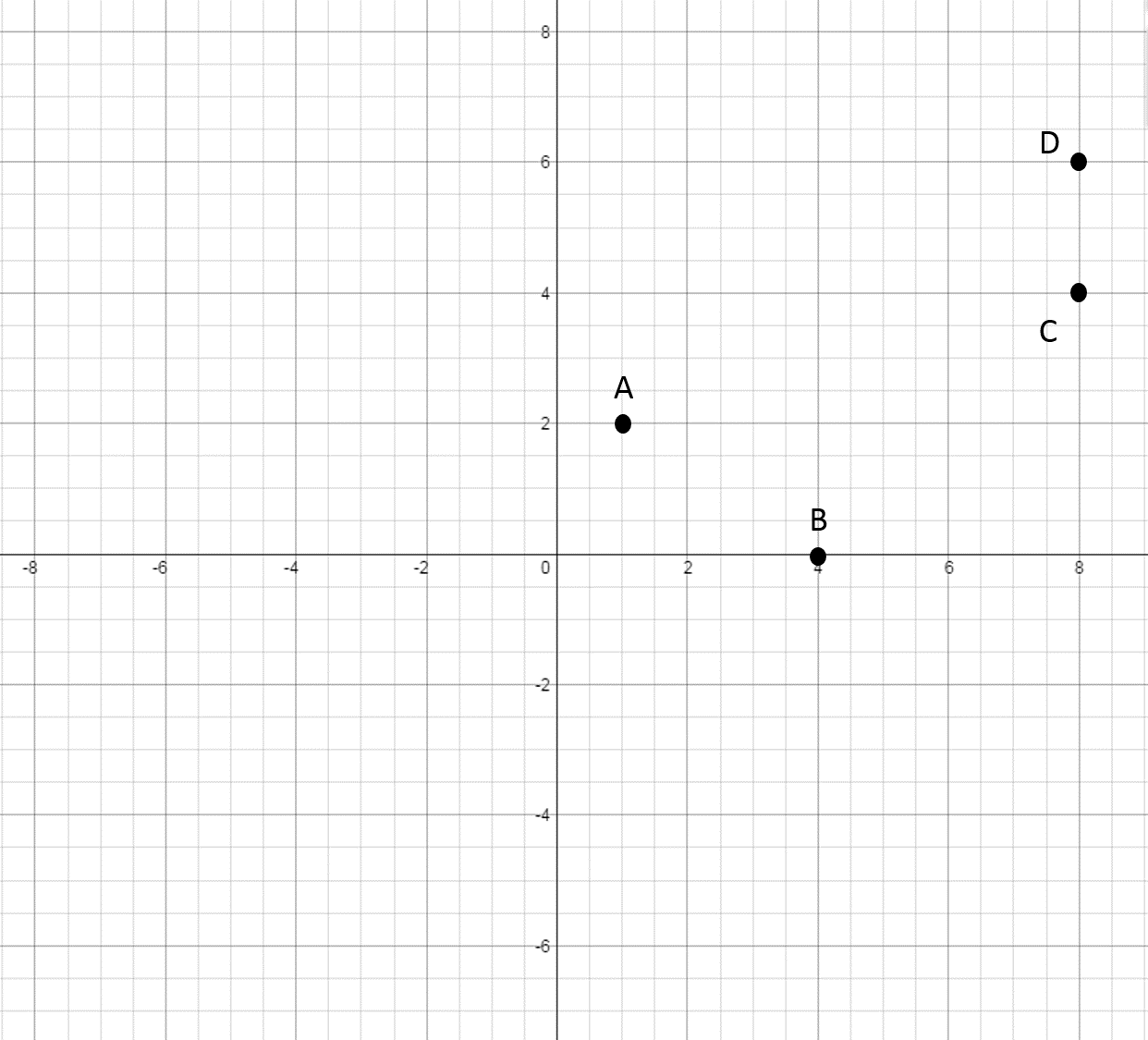Find the point that corresponds to the following ordered pair: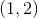Explanation:

In order to get to the point, start at the origin and move rightunit and upunits.

This is point.

### Example Question #8 : Functions And Lines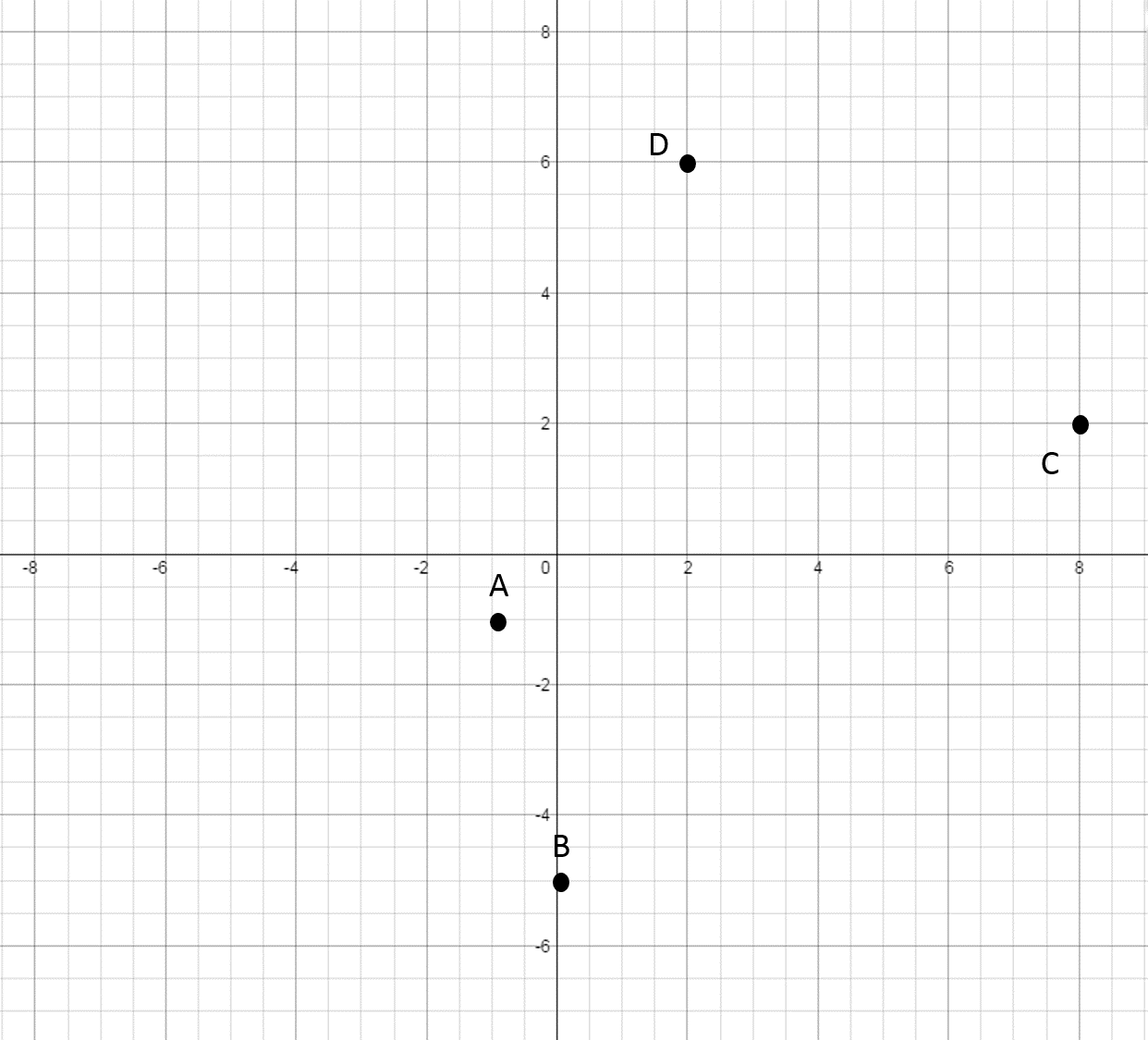Find the point that corresponds to the following ordered pair: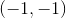Explanation:

In order to get to the point, start at the origin and move leftunit and downunit.

This is point.

### Example Question #9 : Functions And Lines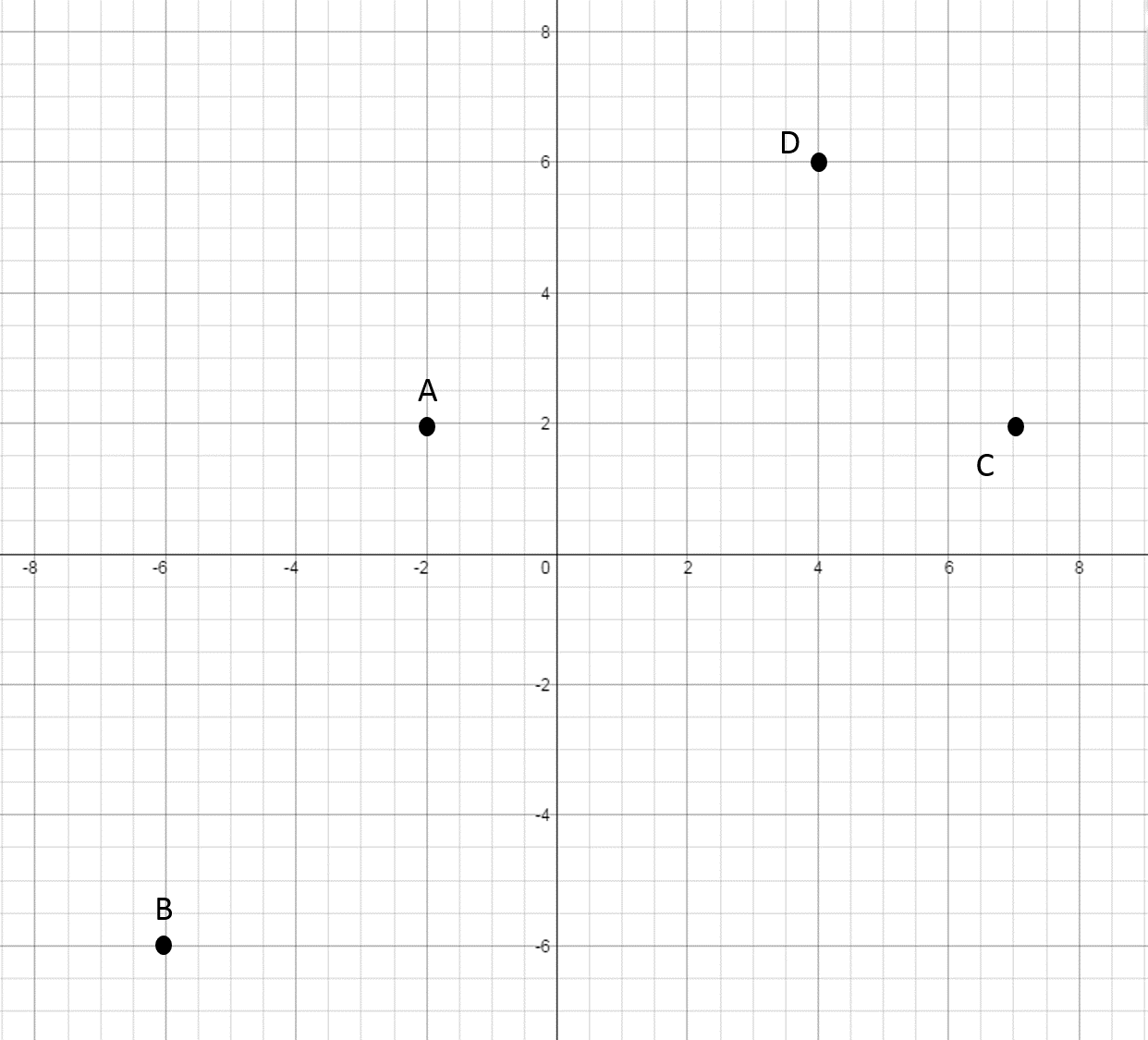Find the point that corresponds to the following ordered pair: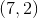Explanation:

In order to get to the point, start at the origin and move rightunits and upunits.

This is point.

← Previous 1 3 4 5

### All Algebra 1 Resources